#### Bernoulli Sampling

Bernoulli sampling, which you request by specifying the METHOD=BERNOULLI option, is an equal probability selection method for which the total sample size is not fixed. PROC SURVEYSELECT performs an independent random selection trial for each of the N sampling units in the input data set by using the constant inclusion probability (sampling rate) that you specify. You can specify a single value of the inclusion probability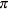to use for all N sampling units, or you can specify separate stratum-level values of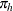to use for the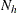units in each stratum.

You provide the inclusion probability (or probabilities) by specifying the SAMPRATE= option. For stratified sampling (which you request with the STRATA statement), you can specify the same sampling rate for each stratum by using the SAMPRATE=r option. Or you can specify different sampling rates for different strata by using the SAMPRATE=(values) or SAMPRATE=SAS-data-set option.

In Bernoulli sampling, the sample size n (number of units selected) is not fixed; it is a random variable that has a binomial distribution with parameters N and. The possible values of n range from 0 to N. The expected value of the sample size is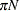(or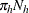for stratified sampling), and the variance of the sample size is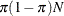.

For Bernoulli sampling, the selection probability is the inclusion probability that you specify by using the SAMPRATE= option. PROC SURVEYSELECT computes the sampling weight as the inverse of the selection probability, which is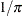. For Bernoulli sampling, the procedure also computes an adjusted sampling weight as the ratio of the total number of sampling units to the actual sample size,(or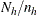for stratified sampling). The joint selection probability for any two distinct units is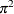. See Särndal, Swensson, and Wretman (1992) for more information.

You can specify the STATS option to include the following information in the OUT= output data set for METHOD=BERNOULLI: total number of sampling units, selection probability, expected sample size, actual sample size, sampling weight, and adjusted sampling weight.